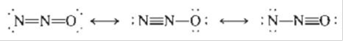# Nitrous oxide (N 2 O) has three possible Lewis structures: Given the following bond lengths, N—N 167 pm N9N 120 pm N:N 110 pm N9O 115 pm N—O 147 pm rationalize the observations that the N—N bond length in N 2 O is 112 pm and that the N—O bond length is 119 pm. Assign formal charges to the resonance structures for N 2 O. Can you eliminate any of the resonance structures on the basis of formal charges? Is this consistent with observation?### Chemistry: An Atoms First Approach

2nd Edition
Steven S. Zumdahl + 1 other
Publisher: Cengage Learning
ISBN: 9781305079243

#### Solutions

Chapter
Section### Chemistry: An Atoms First Approach

2nd Edition
Steven S. Zumdahl + 1 other
Publisher: Cengage Learning
ISBN: 9781305079243
Chapter 3, Problem 104E
Textbook Problem
31 views

## Nitrous oxide (N2O) has three possible Lewis structures:Given the following bond lengths,N—N 167 pmN9N 120 pmN:N 110 pmN9O 115 pmN—O 147 pmrationalize the observations that the N—N bond length in N2O is 112 pm and that the N—O bond length is 119 pm. Assign formal charges to the resonance structures for N2O. Can you eliminate any of the resonance structures on the basis of formal charges? Is this consistent with observation?

Interpretation Introduction

Interpretation: The observation that the NN bond length in N2O is 112pm and that the NO bond length is 119pm to be explained. The elimination of any resonance structure of N2O on the basis of formal charge is to be identified. The consistency of this elimination is to be checked with the given observation.

Concept introduction: The Lewis structure is also known as dot structure. This structure depicts the bonding between atoms and the lone pairs of electrons if exists.

Formal charge is a charge given to an atom with assumption that electrons are shared equally between atoms in all chemical bonds irrespective of their electronegativity.

To determine: The rationalization of the observation that the NN bond length in N2O is 112pm and that the NO bond length is 119pm; if any of the resonance structure of N2O can be eliminated on the basis of formal charge; if this elimination is consistent with the given observation.

### Explanation of Solution

To determine: The rationalization of the observation that the NN bond length in N2O is 112pm and that the NO bond length is 119pm.

Generally, nitrogen atom forms triple bond with other elements and oxygen atom forms two bonds with other elements. The overall bond length of NN is 112pm. Since, the given bond length of NN bond is 110pm and nitrogen atom mostly forms triple bond with other atoms, yet NN and N=N bond also exists. The average of these bond lengths is close to 112pm.

Correspondingly, oxygen atom mostly forms two bonds with other elements. Hence, the bond length of NO falls down to 119pm as it is the average of more stable N=O bond and less stable NO bond.

To determine: If any of the resonance structure of N2O can be eliminated on the basis of formal charge.

The N2O molecule has three resonance structures,

Figure 1

Formula

The formula of formal charge is,

Formal charge =V[N+B2]

Where,

• V is number of valence electrons.
• N is number of non-bonding valence electrons.
• B is total number of bonds shared with other atoms in the given molecule.

The formal charge of first (I) Lewis structure is calculated as,

For central nitrogen (N) atom,

Formal charge =5[0+(12×8)]=+1

For terminal nitrogen (N) atom,

Formal charge =5[4+(

### Still sussing out bartleby?

Check out a sample textbook solution.

See a sample solution

#### The Solution to Your Study Problems

Bartleby provides explanations to thousands of textbook problems written by our experts, many with advanced degrees!

Get Started

Find more solutions based on key concepts
Water from public water systems requires frequent borne testing for microorganisms. is less healthful than bott...

Nutrition: Concepts and Controversies - Standalone book (MindTap Course List)

How does probability relate to spontaneity?

Chemistry for Engineering Students

Calculate the formula mass of sulfuric acid.

Introductory Chemistry: An Active Learning Approach

A particle moves along the xaxis from x = 12.8 m to x = 23.7 m under the influence of a force F=375x3+3.75x whe...

Physics for Scientists and Engineers, Technology Update (No access codes included)

Why did geologists have such strong objections to Wegeners ideas when he proposed them in 1912?

Oceanography: An Invitation To Marine Science, Loose-leaf Versin